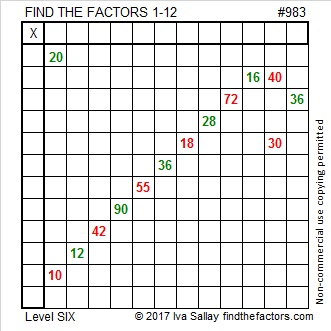# 983 Candy Cane

Candy canes have been a part of the Christmas season for ages. Here’s a candy cane puzzle for you to try. It’s a level 6 so it won’t be easy, but you will taste its sweetness once you complete it. Go ahead and get started!Print the puzzles or type the solution in this excel file: 12 factors 978-985

Here’s some information about prime number 983:

983 is the sum of consecutive prime numbers two different ways:
It is the sum of the seventeen prime numbers from 23 to 97.
It is also the sum of the thirteen prime numbers from 47 to 103.

• 983 is a prime number.
• Prime factorization: 983 is prime.
• The exponent of prime number 983 is 1. Adding 1 to that exponent we get (1 + 1) = 2. Therefore 983 has exactly 2 factors.
• Factors of 983: 1, 983
• Factor pairs: 983 = 1 × 983
• 983 has no square factors that allow its square root to be simplified. √983 ≈ 31.35283

How do we know that 983 is a prime number? If 983 were not a prime number, then it would be divisible by at least one prime number less than or equal to √983 ≈ 31.4. Since 983 cannot be divided evenly by 2, 3, 5, 7, 11, 13, 17, 19, 23, 29 or 31, we know that 983 is a prime number.This site uses Akismet to reduce spam. Learn how your comment data is processed.